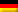The boiling temperature of the mixture can be determined using the Antoine constants on page 250.

According to Dalton (page 256), the following applies at the boiling temperature of the mixture:
p = xB · pB + xT · pT

Calculation of the mass fractions from the mass fractions (p. 52) and the molar masses (pages 110/111 or 131 and 143):

MB = 78,11 g/mol
MT = 92,14 g/mol

Calculation of vapour pressures according to Antoine (p. 250):

Antoine Constants for Benzene:
AB = 6,01762   BB = 1203,331   CB = 219,888
Boiling temperature ϑB = 80,1 °C

Antoine constants for toluene:
AT = 6,07577   BT = 1342,31   CT = 219,187
Boiling temperature: ϑT = 111 °C

With p = xB · pB + xT · pT and

and

for an ambient pressure of p = 1 bar = 100 kPa:

When resolved, this results in a boiling temperature of ϑbZ = 98,6 °C

Dieser Beitrag ist auch verfügbar auf:Deutsch (German)